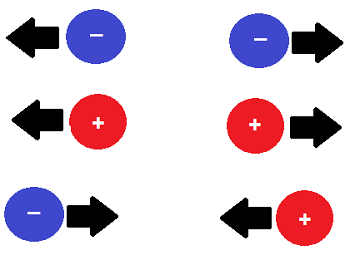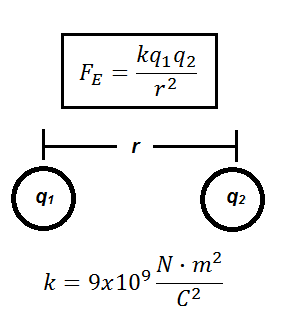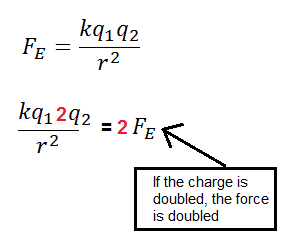# Electromagnetic Force: Definition & Characteristics

Instructor: Betsy Chesnutt

Betsy has a Ph.D. in biomedical engineering from the University of Memphis, M.S. from the University of Virginia, and B.S. from Mississippi State University. She has over 10 years of experience developing STEM curriculum and teaching physics, engineering, and biology.

Electromagnetic forces are important in so many ways! In this lesson, you will learn about different types of electromagnetic forces and how to calculate them.

## What is Electromagnetic Force?

Are you sitting in a chair right now? What is keeping you from falling through the chair all the way to the ground? If you could suddenly shrink and become as small as the atoms in the chair, you would see that the chair, just like all matter, is actually mostly made of empty space with some very tiny, charged particles floating around in it.

It is the interactions between these charges that make the chair feel solid and keep you from falling through it. Electromagnetic force can be used to understand and explain how charged particles, like those in your chair, interact with each other. The electromagnetic force is one of the fundamental forces of the universe, and it affects our reality in some pretty profound ways.

Electromagnetic forces are responsible for holding atoms together in solids and liquids, creating friction forces that allow you to walk across the room, producing light and radio waves, generating electricity, and so much more!There are actually two general types of electromagnetic forces: electrostatic forces and magnetic forces.

## Electrostatic Forces and Coulomb's Law

All electric charges exert electrostatic forces on each other, whether those charges are moving or stationary. Electrostatic forces can cause charges to push away from each other or to be attracted to each other. If two charges are the same (either both positive OR both negative), they will repel each other, but if they are different (one positive and one negative), they will attract each other.How MUCH force will be exerted depends on a couple of things. If the charges get closer together, the force will increase, and if they get farther apart, it will decrease, so the amount of force in inversely proportional to the distance between the charges. The amount of force also depends on the magnitude of the two charges. As the amount of charge goes up, so does the force.

Mathematically, you can calculate the electromagnetic force between any two charges using Coulomb's Law, where k is a constant, q1 and q2 are the two quantity charges (measured in Coulombs) and r is the distance between them (measured in meters):## Using Coulomb's Law

So, what would happen to the force between two charged particles if you double the amount of charge on q1?

According to Coulomb's Law, if you double the charge of one of the particles, then the force will also be doubled.To unlock this lesson you must be a Study.com Member.

### Register to view this lesson

Are you a student or a teacher?